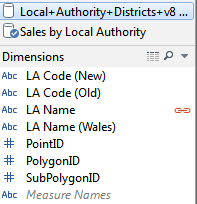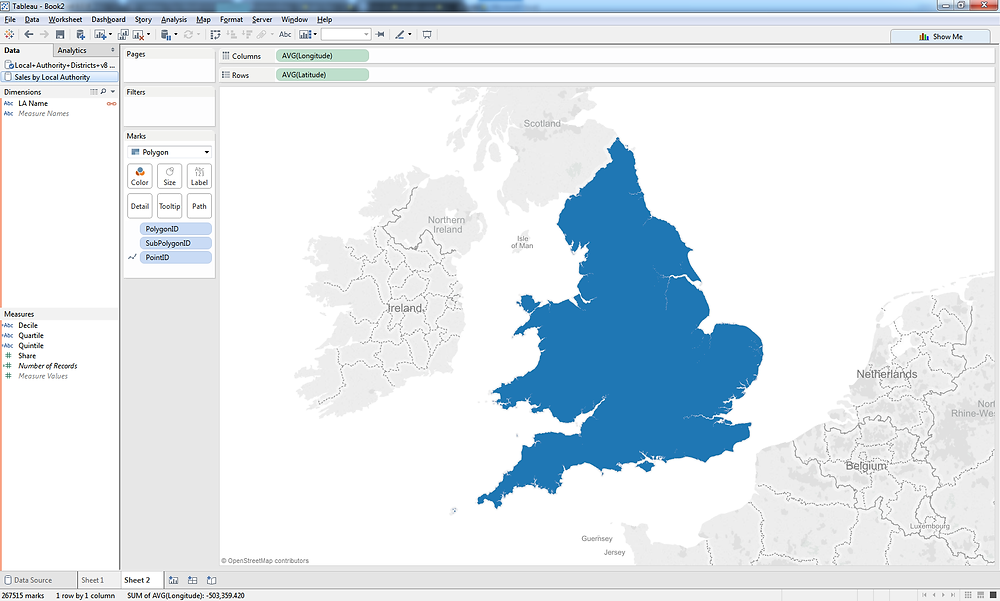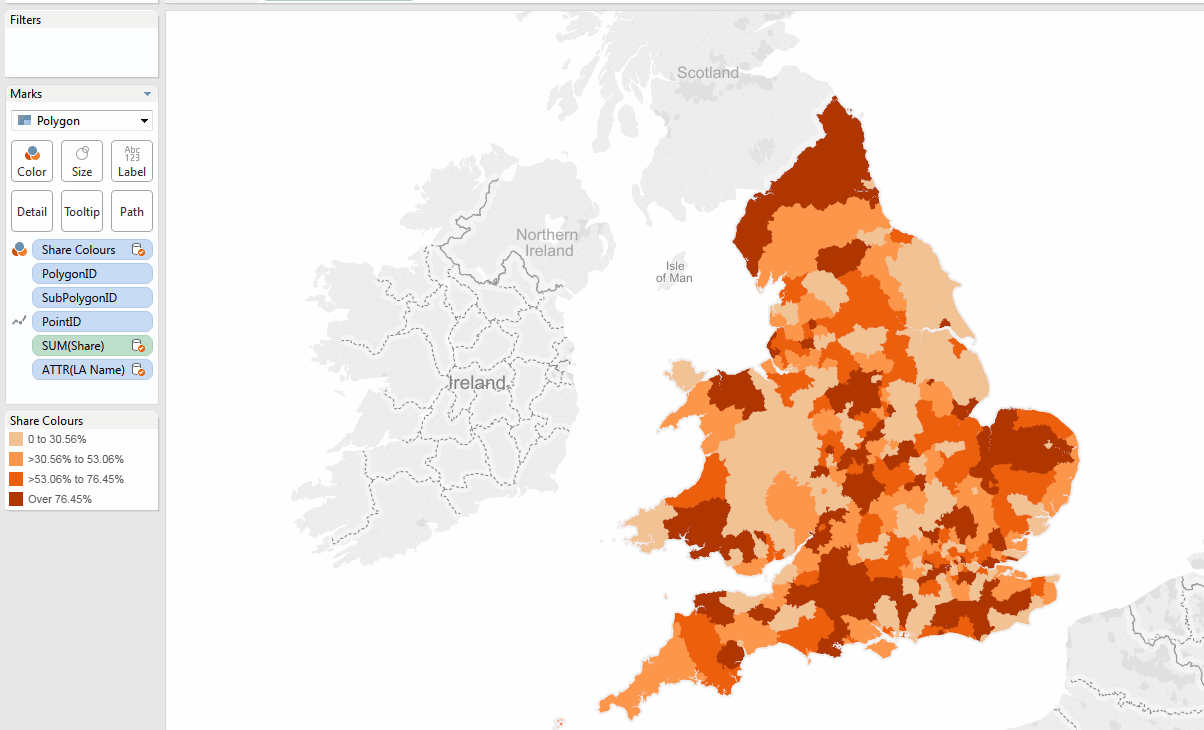Search

# TableauTips: Mapping using Quantiles

Tableau Public has made March 2016 #TableauTipsMonth and I hope to contribute with a few tips I’ve learned and use often. Here’s the 1st one:

## Mapping using Quantiles

We can colour maps easily in Tableau, but I’m not quite sure what the algorithm behind the gradient is, and often I am asked to produce maps using quantiles.

The idea being that you use your rows of data and distribute them in equal bands, additionally the ability to label each quantile and use the highlight function makes this a handy trick to have up your sleeve. I saw the original calculation by Joe Mako which allowed me to get started and develop from there.

I started by taking Information Lab’s polygon data of Local Authorities in the England and Wales.

After creating a polygon map, which you can see instructions on the same website, I created some random share data per row matching LA Name. If you ever in doubt about how to randomise data, maybe for a workbook that you want to share on Tableau forum for help, I always use the excel function randbetween, in this case I wanted 4 decimals so I used the formula below.

=RANDBETWEEN(1000,10000)/10000

Once I’ve added the data to Tableau, I create a calculated field:

IF INDEX() <= INT((25/100)*(SIZE()+1)) THEN “1st” ELSEIF INDEX() <= INT((50/100)*(SIZE()+1)) THEN “2nd” ELSEIF INDEX() <= INT((75/100)*(SIZE()+1)) THEN “3rd” ELSEIF INDEX() <= INT((100/100)*(SIZE()+1)) THEN “4th” END

Joe would probably explain this a thousand times better than I can, but the idea is that you change the INT((25/100) to fit the size of split you want. The above is an example for a Quartile, below you have a Quintile:

IF INDEX() <= INT((20/100)*(SIZE()+1)) THEN “1st” ELSEIF INDEX() <= INT((40/100)*(SIZE()+1)) THEN “2nd” ELSEIF INDEX() <= INT((60/100)*(SIZE()+1)) THEN “3rd” ELSEIF INDEX() <= INT((80/100)*(SIZE()+1)) THEN “4th” ELSEIF INDEX() <= INT((100/100)*(SIZE()+1)) THEN “5th” END

and a Decile

IF INDEX() <= INT((10/100)*(SIZE()+1)) THEN “1st” ELSEIF INDEX() <= INT((20/100)*(SIZE()+1)) THEN “2nd” ELSEIF INDEX() <= INT((30/100)*(SIZE()+1)) THEN “3rd” ELSEIF INDEX() <= INT((40/100)*(SIZE()+1)) THEN “4th” ELSEIF INDEX() <= INT((50/100)*(SIZE()+1)) THEN “5th” ELSEIF INDEX() <= INT((60/100)*(SIZE()+1)) THEN “6th” ELSEIF INDEX() <= INT((70/100)*(SIZE()+1)) THEN “7th” ELSEIF INDEX() <= INT((80/100)*(SIZE()+1)) THEN “8th” ELSEIF INDEX() <= INT((90/100)*(SIZE()+1)) THEN “9th” ELSEIF INDEX() <= INT((100/100)*(SIZE()+1)) THEN “10th” END

I have labelled them 1st to x as I find it easier to understand, the 1st being the lowest quantile. This will become useful later on.

Next step bring my LA Name, Share as a table and very important, sort in ascending order.

This is what you should be looking at:From here I start making notes of the intersections between each band.

0-0.3056>0.3056 – 0.5306 (you are bound to have situations where your bands cross as you don’t have even numbers. You’ll have to decide where you want your bands to cross. It will depend on the analysis.)>0.5306 – 0.7645After you have identified all the intersections is time to create your IF statement, I’ve named it “Share Colours”.

IF [Share] > 0.7645 then “Over 76.45%” ELSEIF [Share] > 0.5306 then “>53.06% to 76.45%” ELSEIF [Share] > 0.3056 then “>30.56% to 53.06%” ELSEIF [Share] > 0 then “0 to 30.56%” END

Go back to the map you have created and blend the data on LA Name.For more about data blending check this video tutorial: Blending in TableauAfter which we drop our “Share Colours” field into the colour shelf and this is the result:Finally change the colours as you need, either gradient or distinguished and don’t forget to use the highlight tool to illustrate the point you are trying to make.I hope you have found this useful and feel free to leave comments or questions below. I’ve also published the workbook to Tableau Public in case you need it.

Mapping with Quantiles Workbook

David

See All